The AUTOREG Procedure
 TEST Statement

The AUTOREG procedure supports a TEST statement for linear hypothesis tests. The syntax of the TEST statement is

TEST equation , ..., equation / option ;

The TEST statement tests hypotheses about the covariates in the model that are estimated by the preceding MODEL statement. The AR, GARCH, and HETERO parameters are also supported in the TEST statement. Each equation specifies a linear hypothesis to be tested. If more than one equation is specified, the equations are separated by commas.

Each test is written as a linear equation composed of constants and parameter names. Refer to parameters by the name of the corresponding regressor variable. Each name used in the equation must be a regressor in the preceding MODEL statement. Use the keyword INTERCEPT to refer to the intercept parameter in the model. See the section OUTEST= Data Set for the names of these parameters.

You can specify the following options in the TEST statement:

TYPE=value

specifies the test statistics to use. The default is TYPE=F. The following values for TYPE= option are available:

F

produces an F test. This option is supported for all models specified in MODEL statement.

WALD

produces a Wald test. This option is supported for all models specified in MODEL statement.

LM

produces a Lagrange multiplier test. This option is supported only when the GARCH= option is specified (for example, when there is a statement like MODEL Y = C D I / GARCH=(Q=2)).

LR

produces a likelihood ratio test. This option is supported only when the GARCH= option is specified (for example, when there is a statement like MODEL Y = C D I / GARCH=(Q=2)).

ALL

produces all tests applicable for a particular model. For non-GARCH-type models, only F and Wald tests are output. For all other models, all four tests (LR, LM, F, and Wald) are computed.

The following example of a TEST statement tests the hypothesis that the coefficients of two regressors A and B are equal:

```model y = a b c d;
test a = b;
```

To test separate null hypotheses, use separate TEST statements. To test a joint hypothesis, specify the component hypotheses on the same TEST statement, separated by commas.

For example, consider the following linear model: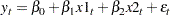The following statements test the two hypotheses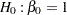and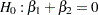:

```model y = x1 x2;
test intercept = 1;
test x1 + x2 = 0;
```

The following statements test the joint hypothesis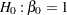and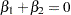:

```model y = x1 x2;
test intercept = 1, x1 + x2 = 0;
```

To illustrate the TYPE= option, consider the following examples.

```model Y = C D I / garch=(q=2);
test C + D = 1;
```

The proceding statements produce only one default test, the F test.

```model Y = C D I / garch=(q=2);
test C + D = 1 / type = LR;
```

The proceding statements produce one of four tests applicable for GARCH-type models, the likelihood ratio test.

```model Y = C D I / nlag = 2;
test C + D = 1 / type = LM;
```

The proceding statements produce the warning and do not output any test because the Lagrange multiplier test is not applicable for non-GARCH models.

```model Y = C D I / nlag=2;
test C + D = 1 / type = ALL;
```

The proceding statements produce all tests that are applicable for non-GARCH models (namely, the F and Wald tests). The TYPE= prefix is optional. Thus the test statement in the previous example could also have been written as:

```test C + D = 1 / ALL;
```

The following example shows how to test AR, GARCH, and HETERO parameters:

```model y = a b / nlag=2 garch=(p=2,q=3,mean=sqrt);
hetero c d;
test _A_1=0,_AH_2=0.2,_HET_2=1,_DELTA_=0.1;
```Previous Page | Next Page | Top of Page